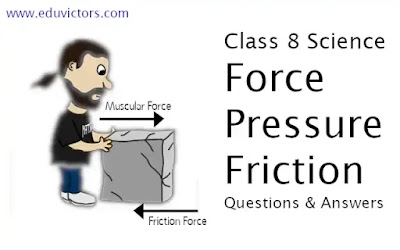## Force, Pressure and Friction

CBSE Class 8 - Science

Q1: Why do porters place on their heads a round piece of cloth?

Round piece of cloth increases contact surface areas, thus reduce the pressure.

Q2: It is convenient to pull the luggage fitted with rollers, why?

Answer: Because rollers reduce friction. Rolling friction is much less than sliding friction.

Q3: lf we apply a force on a body and the body is still in rest. Is there any frictional force acting on the body? If yes then how?

Answer: Yes. Static friction is acting on it in opposite direction to the applying force. Thus, resultant force is zero.

Q4:  Sliding friction is less than static friction, how? Explain by an example.

Answer:  Friction is due to interlocking between the two contact surfaces. In case of sliding surfaces, there is less interlocking, hence value of sliding friction is less.

Q5:  Why is it difficult to walk a smooth and wet floor? |

Answer: Because value of frictional force become less. The coating of water reduces the friction and the foot cannot make a tighter grip on the floor, it starts slipping.

Q6:  What is pressure?

Answer: Force acting on a unit area of surface is called pressure. Its SI unit is Pascal (Pa)

Q7: What do you understand by ‘static friction’?

Answer:  When we try to move a body by applying force and body is still in rest, the friction on the body is called ‘static friction’.

Q8: What do you understand by ‘contact force’?

Answer: When a force can be applied on an object only when it is in contact with the object.

Q9: Write down the name of two forces which are ‘Non contact forces’?

Answer:  Electrostatic force and gravitational force.

Q10:  ‘How do you define ‘Atmospheric pressure’?

Answer: The pressure exerted by the air around us is called atmospheric pressure.

Q11: Name the factors, on which friction force on an object in a fluid depends?

Answer: The factors, on which friction force on an object in a fluid depends are:
- Velocity of object with respect to fluid
- Shape of the object .
- Nature of the fluid

Q12: Mention the necessary conditions for a force to come into play.

Answer: Interaction between two objects is required for a force to come into play. The interaction of one object with other results in a force between two objects but no physical contact is necessary.

Q13: Explain brief about muscular force along with the help of an example.

Answer: The force exerted by the muscles of arms or legs or any other body part in order to do some work is called muscular force.

Kicking a football, pushing a car are some examples.

Q14: A sharp axe cuts the long earlier than a blunt axe. Explain why.

Answer: A sharp axe has a sharp edge which means edge has a minimum area as compared to the rest of the axe. As the area is small, the pressure is large and thus easier to cut trees. Whereas blunt axe has larger edge thus exerting less pressure and hence it takes time to cut trees.

Q15: Name the device used to measure atmospheric pressure.

Q16: As we go to high altitude the atmospheric pressure goes on decreasing. Give the reason.

Answer: At higher altitude, the number of air molecules per unit area decreases. As a result, the force exerted by the air molecules decreases resulting in low pressure at higher altitudes.

Q17: Why can a camel walk in deserts easily?

Answer: Camels have padded feet that imply that its feet have more area. So when they walk on the sand, their body exerts less pressure on the sand which prevents them from sinking and thus helps them to walk.

Q18: Is gravity a property of the earth alone?

Answer: No. In fact, every object in the universe whether small or large, exerts a force on every other object. This force is also known as the gravitational force.

Q19: Why are the walls of the dams made stronger and thicker at the bottom than at the top?

Answer: The walls of the dams are made stronger and thicker at the bottom than at the top of the dam to withstand the huge lateral pressure of water at the bottom.

Q20: Why do astronauts wear a special dress to go into the space?

Answer: The blood pressure inside our body would need air pressure outside to keep us safe.
When we go above the earth’s atmosphere, the pressure outside is very less. But, the pressure inside our body is very high. Due to this our body will burst. So, to avoid this astronauts wear a special dress.

Ch 11 - Force and Pressure (Q & A)
Ch11 - Force and Pressure (MCQs)
Ch 11 - Force (What is Inertia?)
Ch 11 - Force and Pressure (Assignment Sheet)
Ch 11 - Force and Pressure (Worksheet)

Ch 12 - Friction
Ch 12 - Friction (MCQs-1)
Ch 12 - Friction (MCQs-2)
Ch12 - Friction (Assignment)
Ch 12 - Friction (Worksheet)
Ch 12 - Friction (Worksheet-2018-19)